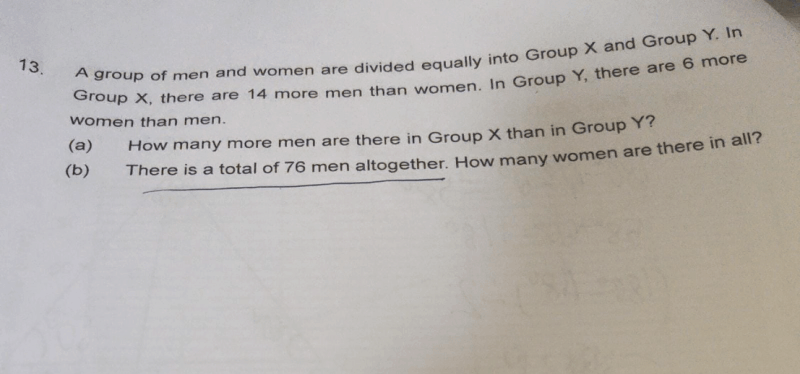# QuestionGroup X

Men -> M(x)

Women -> W(x)

Given: 14 more men than women -> W(x) = M(x) – 14

Group Y

Men -> M(y)

Women -> W(y)

Given: 6 more women than men -> W(y) = M(y) + 6

The 2 groups have equal number of people

M(x) + W(x) = M(y) + W(y)

M(x) + M(x) – 14 = M(y) + M(y) + 6

2M(x) – 14 = 2M(y) + 6

2M(x) – 2M(y) = 20

M(x) – M(y) = 10

(a) Group X has 10 more men than Group Y

M(x) – M(y) = 10

M(x) = M(y) + 10

Given: there are a total of 76 men

M(x) + M(y) = 76

M(y) + 10 + M(y) = 76

2M(y) = 66

M(y) = 33

M(x) = 76 – 33 = 43

W(x) = 43 – 14 = 29

W(y) = 33 + 6 = 39

(b) There are (29 + 39) = 68 women in all

0 Replies 0 Likes